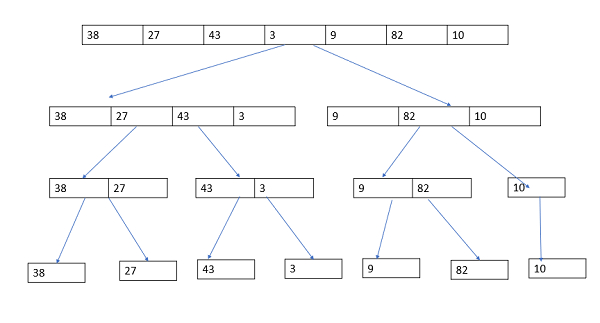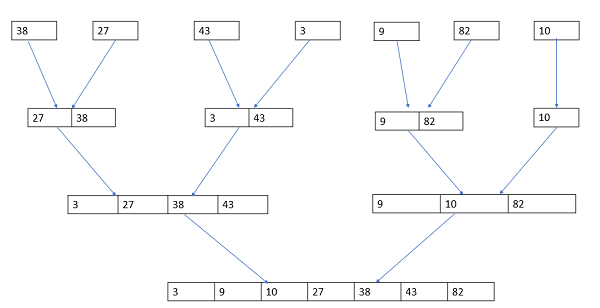# Explain the merge sort technique in C language

Sorting is the process of arranging the elements either in ascending (or) descending order.

## Types of sorting

C language provides five sorting techniques, which are as follows −

• Bubble sort (or) Exchange Sort
• Selection sort
• Insertion sort(or) Linear sort
• Quick sort (or) Partition exchange sort
• Merge Sort (or) External sort

## Merge sort

Merge sort is a divided and conquer method. It divides an array in two half’s, conquer recursively and merge (combine).

Let’s consider an example which is given below −

Take an unsorted array and apply merge sort technique to sort the array.

38, 27, 43, 3, 9, 82, 10Now, combine an array by sorting as shown below −## Example

Following is the C program to sort the elements by using the merge sort technique −

Live Demo

#include <stdio.h>
#define max 10
int a = { 10, 14, 19, 26, 27, 31, 33, 35, 42, 44, 0 };
int b;
void merging(int low, int mid, int high) {
int l1, l2, i;
for(l1 = low, l2 = mid + 1, i = low; l1 <= mid && l2 <= high; i++) {
if(a[l1] <= a[l2])
b[i] = a[l1++];
else
b[i] = a[l2++];
}
while(l1 <= mid)
b[i++] = a[l1++];
while(l2 <= high)
b[i++] = a[l2++];
for(i = low; i <= high; i++)
a[i] = b[i];
}
void sort(int low, int high) {
int mid;
if(low < high) {
mid = (low + high) / 2;
sort(low, mid);
sort(mid+1, high);
merging(low, mid, high);
} else {
return;
}
}
int main() {
int i;
printf("List before sorting");
for(i = 0; i <= max; i++)
printf("%d ", a[i]);
sort(0, max);
printf("List after sorting");
for(i = 0; i <= max; i++)
printf("%d ", a[i]);
}

## Output

When the above program is executed, it produces the following output −

List before sorting
10 14 19 26 27 31 33 35 42 44 0
List after sorting
0 10 14 19 26 27 31 33 35 42 44